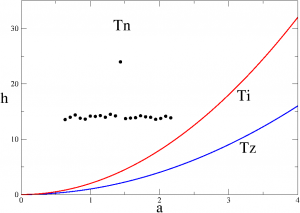# ISC meeting – Diffusion Processes

An ISC-meeting on diffusion processes took place in Sesto Fiorentino (Florence) on Sept. 27th and 28th.
It was the occasion to highlight the variety of ISC research fields where diffusion plays a relevant role:

On any lattice site we have a mass $$a_i$$ and an energy $$h_i = a_i^2$$, which may exchange matter by conserving
the total mass and energy.

• At $$T=0$$ the system is frozen and uniform
• At $$T=infty$$ the mass are Poissonian-distributed
• At $$T$$ < $$0$$, localized excitations (breathers) appearIf brownian motion has been a milestone to understand the atomistic nature of matter, diffusion processes are so widespread because diffusion is one of the main “way of transport”, as we learn from the kinetic theory. In addition, a couple of relevant progresses make diffusion an active and modern research topic.

On the one hand, the variety of processes where standard diffusion must give way to anomalous diffusion (Lepri): here, the average, travelled square distance does not scale linearly with time. On the other hand, the variety of different environments where diffusion takes place: from confined, artificial structures (Cecconi, Del Rosso) to brain tissues (Capuani-Gabrielli).